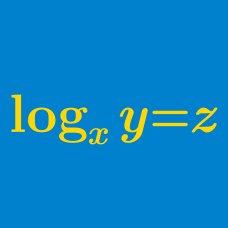Algebra

# Logarithmic Functions Problem Solving

Let $w, x, y, z$ be positive reals greater than 1, and

$\log_x w = 24, \log_y w = 40, \log_{xyz} w = 12 .$

Evaluate $\log_z w$.

The only real solution of the equation $\ln x + \ln (x^2-34)=\ln 72$ can be uniquely written in the form $a+\sqrt{b}$ for some integers $a$ and $b$. Find $a+b$.

Given that $\log_{10}2=0.3010$, $\log_{10}3=0.4771$ and $\log_{10}7=0.8451$, let $a$ be the number of digits in $3^{62}$. If $b$ is the first digit fromthe left of $3^{62}$, what is $a+b$?

Let $N$ be the number of times that the first digit of $2^n$ is $1,$ with $n$ being an integer such that $0\leq n \leq 10^{10}$. What are the last three digits of $N?$

You may use the fact that
$0.3010299956639811952 < \log_{10} 2 < 0.3010299956639811953$

Evaluate $\lfloor \log_{8} 1 \rfloor + \lfloor \log_{8} 2 \rfloor + \lfloor \log_{8} 3 \rfloor + \cdots + \lfloor \log_{8} 154 \rfloor.$

Details and assumptions

Greatest Integer Function / Floor Function: The function $\lfloor x \rfloor: \mathbb{R} \rightarrow \mathbb{Z}$ refers to the greatest integer smaller than or equal to $x$. For example, $\lfloor 2.3 \rfloor = 2$ and $\lfloor -5 \rfloor = -5$.

×